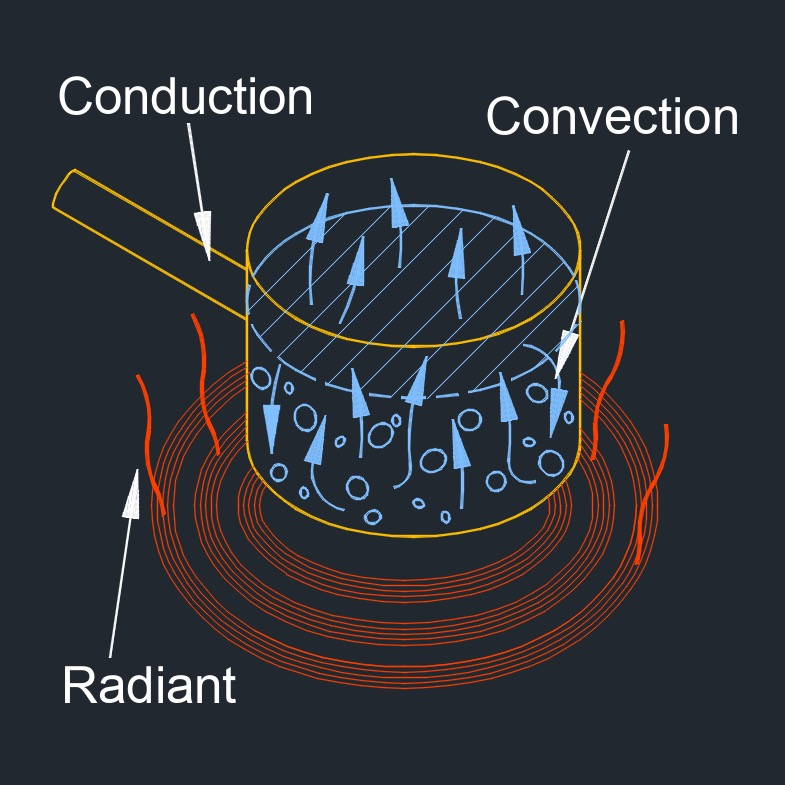# Heat Capacity

on . Posted in ThermodynamicsHeat capacity, abbreviated as C or $$c_p$$, is the amount of enerigy required to increase the temperature of a substance by 1°C.  The heat gain or loss results in a change in temperature and the state and performance of work.

## Heat capacity formula

$$\large{ C = \frac {\Delta Q} { \Delta T } }$$

Symbol English Metric
$$\large{ C }$$ = heat capacity $$\large{\frac{Btu}{F}}$$ $$\large{\frac{kJ}{K}}$$
$$\large{ \Delta Q }$$ = heat transfered amount $$\large{\frac{Btu}{hr}}$$ $$\large{ W }$$
$$\large{ \Delta T }$$ = temperature differential $$\large{ F }$$ $$\large{ K }$$